Next: Unequal Subcycled to Non-Subcycled Up: Multi-Time Step Subcycling Results Previous: Multi-Time Step Subcycling Results   Contents

## Equal Subcycled to Non-Subcycled Region Ratio of 1:1

We first test subcycling on an equal distribution of non-subcycled and subcycled nodes, i.e. a region ratio of , with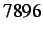nodes,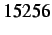edges andvolumetric elements. Selecting a random node ahead of the initial notch, we plot its nodal displacements in Figure 4.2 for the various subcycling parameters. From this figure we can see that subcycled solutions are very close to the reference solution, although their accuracies decrease with an increasing subcycling parameter.

The timing results for the internal and cohesive force subroutines, the main loop and the overall solution are presented in Table 4.5. As expected, the time for the internal force calculations decreases as the subcycling parameter grows, although for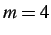, the cost resulting from the cohesive forces and main solution loop offsets the savings of the internal forces - aloss.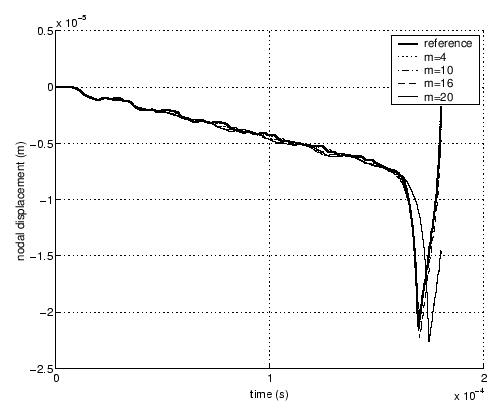Table 4.1: Timing results (in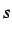) for a problem with an equal region ratio () with subcycling parameters of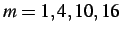and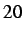.
 Subroutine Reference Case [s], m = 1 m = 4 m = 10 m = 16 m = 20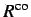424.13 628.72 503.81 479.24 469.56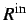733.89 472.52 300.37 256.66 238.05427.29 652.91 499.89 469.59 439.78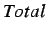1628.54 1811.59 1359.70 1259.68 1201.80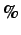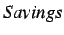-11% 17% 23% 26%Next: Unequal Subcycled to Non-Subcycled Up: Multi-Time Step Subcycling Results Previous: Multi-Time Step Subcycling Results   Contents
Mariusz Zaczek 2002-10-13